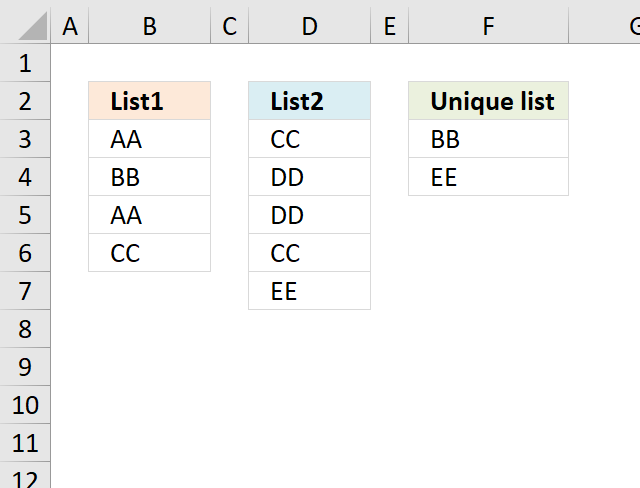Author: Oscar Cronquist Article last updated on November 17, 2018

I read an article Merging Lists To A List Of Distinct Values at CPearson. The article describes code that you can use to merge two lists into a third list and prevent duplicate entries in the resulting list, using VBA to create a macro.

Here is a solution to create a unique list from two columns without using VBA.

The picture below shows the unique list in column F.The following formula returns values that are unique from two columns combined. Unique values are values that only exist once in both columns.

Formula in cell F3:

=IFERROR(LOOKUP(2, 1/((COUNTIF(F2:\$F\$2, \$B\$3:\$B\$6)+COUNTIF(\$D\$3:\$D\$7, \$B\$3:\$B\$6)+COUNTIF(\$B\$3:\$B\$6, \$B\$3:\$B\$6))=1),\$B\$3:\$B\$6), LOOKUP(2,1/((COUNTIF(F2:\$F\$2, \$D\$3:\$D\$7)+COUNTIF(\$B\$3:\$B\$6, \$D\$3:\$D\$7)+COUNTIF(\$D\$3:\$D\$7, \$D\$3:\$D\$7))=1), \$D\$3:\$D\$7))

### Explaining formula in cell F3

This formula may seem big, however, it is actually two smaller formulas combined. If you want to see the calculation steps then go to tab "Formulas" on the ribbon and click "Evaluate Formula" button, then click "Evaluate" button to move to next step in the calculation.

#### Step 1 - Prevent duplicate values

The COUNTIF function is really helpful in this situation, it counts the values in order to display unique values from two columns combined.

COUNTIF(F2:\$F\$2, \$B\$3:\$B\$6)

becomes

COUNTIF("Unique list", {"AA";"BB";"AA";"CC"})

and returns {0;0;0;0}.

#### Step - 2 - Count values in List1 against List 2

COUNTIF(\$D\$3:\$D\$7, \$B\$3:\$B\$6)

becomes

COUNTIF({"CC";"DD";"DD";"CC";"EE"}, {"AA";"BB";"AA";"CC"})

and returns {0;0;0;2}. This tells us that only "CC" has a duplicate in List2.

#### Step 3 - Count values in List1

The following COUNTIF formula identifies unique values in cell range \$B\$3:\$B\$6

COUNTIF(\$B\$3:\$B\$6, \$B\$3:\$B\$6)

becomes

COUNTIF({"AA";"BB";"AA";"CC"}, {"AA";"BB";"AA";"CC"})

and returns

{2;1;2;1}.

#### Step 4 - Add arrays

(COUNTIF(F2:\$F\$2, \$B\$3:\$B\$6)+COUNTIF(\$D\$3:\$D\$7, \$B\$3:\$B\$6)+COUNTIF(\$B\$3:\$B\$6, \$B\$3:\$B\$6))

becomes

{0;0;0;0} + {0;0;0;2} + {2;1;2;1}

equals

{2;1;2;3}.

#### Step 5 - Check if value in array is equal to 1

(COUNTIF(F2:\$F\$2, \$B\$3:\$B\$6)+COUNTIF(\$D\$3:\$D\$7, \$B\$3:\$B\$6)+COUNTIF(\$B\$3:\$B\$6, \$B\$3:\$B\$6))=1

becomes

{2;1;2;3}=1

and returns

{FALSE;TRUE;FALSE;FALSE}.

#### Step 6 - Divide 1 with array

1/((COUNTIF(F2:\$F\$2, \$B\$3:\$B\$6)+COUNTIF(\$D\$3:\$D\$7, \$B\$3:\$B\$6)+COUNTIF(\$B\$3:\$B\$6, \$B\$3:\$B\$6))=1)

becomes

1/({FALSE;TRUE;FALSE;FALSE})

and returns

{#DIV/0!;1;#DIV/0!;#DIV/0!}

#### Step 7 - Return value based on array

LOOKUP(2, 1/((COUNTIF(F2:\$F\$2, \$B\$3:\$B\$6)+COUNTIF(\$D\$3:\$D\$7, \$B\$3:\$B\$6)+COUNTIF(\$B\$3:\$B\$6, \$B\$3:\$B\$6))=1),\$B\$3:\$B\$6)

becomes

LOOKUP(2, {#DIV/0!;1;#DIV/0!;#DIV/0!},\$B\$3:\$B\$6)

becomes

LOOKUP(2, {#DIV/0!;1;#DIV/0!;#DIV/0!},{"AA";"BB";"AA";"CC"})

and returns "BB" in cell F3.

#### Step 8 - Return values from List 2

When the first formula returns errors the IFERROR function directs to the next formula. The next formula is exactly the same as the first formula except that it gets values from List 2.

=IFERROR(formula1, formula2)

### Download excel sample file for this tutorial

unique list from two columnsv2.xlsx